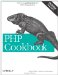# Recipe 2.12. Calculating Trigonometric Functions

#### 2.12.1. Problem

You want to use trigonometric functions, such as sine, cosine, and tangent.

#### 2.12.2. Solution

PHP supports many trigonometric functions natively: sin( ), cos( ), and tan( ):

`\$cos = cos(2.1232);`

You can also use their inverses: asin( ), acos( ), and atan( ):

`\$atan = atan(1.2);`

#### 2.12.3. Discussion

These functions assume all angles are in radians, not degrees. (See Recipe 2.13 if this is a problem.)

The function atan2( ) takes two variables \$x and \$y, and computes atan(\$x/\$y). However, it always returns the correct sign because it uses both parameters when finding the quadrant of the result.

For secant, cosecant, and cotangent, you should manually calculate the reciprocal values of sin( ), cos( ), and tan( ):

`\$n = .707; \$secant    = 1 / sin(\$n); \$cosecant  = 1 / cos(\$n); \$cotangent = 1 / tan(\$n);`

You can also use hyperbolic functions: sinh( ), cosh( ), and tanh( ), plus, of course, asin( ), acosh( ), and atanh( ). The inverse functions, however, aren't supported on Windows.

Recipe 2.13 for how to perform trig operations in degrees, not radians; documentation on sin( ) at http://www.php.net/sin, cos( ) at http://www.php.net/cos, tan( ) at http://www.php.net/tan, asin( ) at http://www.php.net/asin, acos( ) at http://www.php.net/acos, atan( ) at http://www.php.net/atan, and atan2( ) at http://www.php.net/atan2.PHP Cookbook: Solutions and Examples for PHP Programmers
ISBN: 0596101015
EAN: 2147483647
Year: 2006
Pages: 445

Similar book on Amazon